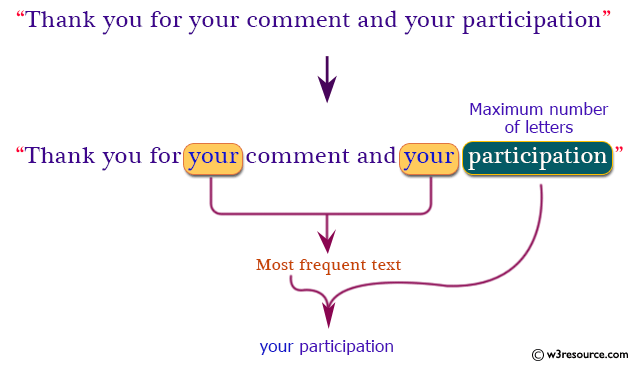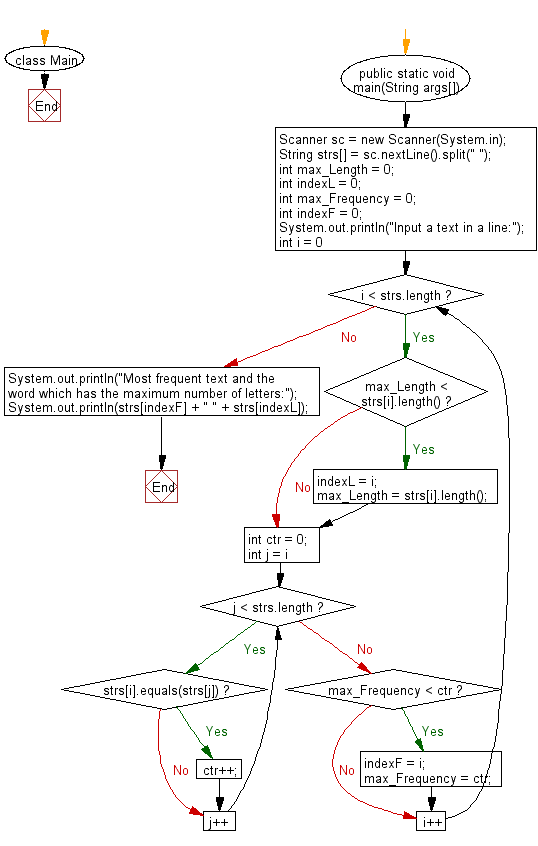# Java Exercises: Reads a text and prints two words

## Java Basic: Exercise-227 with Solution

Write a Java program which reads a text (only alphabetical characters and spaces.) and prints two words. The first one is the word which is arise most frequently in the text. The second one is the word which has the maximum number of letters.

Note: A word is a sequence of letters which is separated by the spaces.

Input:

A text is given in a line with following condition:
a. The number of letters in the text is less than or equal to 1000.
b. The number of letters in a word is less than or equal to 32.
c. There is only one word which is arise most frequently in given text.
d. There is only one word which has the maximum number of letters in given text.

Pictorial Presentation:Sample Solution:

Java Code:

``````import java.util.Scanner;
class Main
{
public static void main(String args[])
{
Scanner sc = new Scanner(System.in);

String strs[] = sc.nextLine().split(" ");
int max_Length = 0;
int indexL = 0;
int max_Frequency = 0;
int indexF = 0;
System.out.println("Input a text in a line:");
for (int i = 0; i < strs.length; i++)
{
if (max_Length < strs[i].length())
{
indexL = i;
max_Length = strs[i].length();
}
int ctr = 0;
for (int j = i; j < strs.length; j++)
{
if (strs[i].equals(strs[j]))
{
ctr++;
}
}
if (max_Frequency < ctr)
{
indexF = i;
max_Frequency = ctr;
}
}
System.out.println("Most frequent text and the word which has the maximum number of letters:");
System.out.println(strs[indexF] + " " + strs[indexL]);
}
}
```
```

Sample Output:

```Thank you for your comment and your participation.
Input a text in a line:
Most frequent text and the word which has the maximum number of letters:
```

Flowchart:Java Code Editor:

What is the difficulty level of this exercise?

﻿

## Java: Tips of the Day

Parsing dates:

```import java.io.*;
import java.util.*;
import java.text.*;

String s = "2001/09/23 14:39";

SimpleDateFormat formatter = new SimpleDateFormat ("yyyy/MM/dd H:mm");
Date d = formatter.parse(s, new ParsePosition(0));
```